Please start any new threads on our new site at https://forums.sqlteam.com. We've got lots of great SQL Server experts to answer whatever question you can come up with.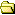All Forums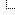General SQL Server Forums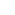Script Library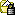Time in Analog

Author  Topic

ditch
Master Smack Fu Yak Hacker

1466 Posts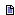Posted - 2004-03-14 : 10:18:35 I was bored, so I tried it.It's not perfect (The results panes fonts mess with it a bit).Best viewed if your QA's font is set to one of the Courier fonts and the results are viewed as text rather than grid.I still want to get it right without using any cursors at all.I will attempt this later.Maybe someone else can improve on this though.Here it is:--**********************************************************CREATE PROCEDURE AnalogTime ASSET NOCOUNT ONCREATE TABLE #ClockTimeDeg(Radius INT, ClockTimeHRS DECIMAL(18, 3), ClockTimeMin INT, Deg DECIMAL(18, 8), XCoOrd DECIMAL(18, 8), YCoOrd DECIMAL(18, 8), DISPLAY VARCHAR(2));DECLARE @Mins INTDECLARE @Hrs DECIMAL(18, 3)DECLARE @Deg DECIMAL(18,8)DECLARE @XCoOrd INTDECLARE @YCoOrd INTDECLARE @CurXCoOrd INTDECLARE @CurYCoOrd INTDECLARE @PrevYCoOrd INTDECLARE @Radius DECIMAL(18, 8)DECLARE @InsString VARCHAR(1000)DECLARE @Display VARCHAR(2)DECLARE @CurrKey INTDECLARE @PrevKey INTDECLARE @CurrTime DATETIMEDECLARE @HrsVal DECIMAL(18, 8)DECLARE @MinsVal INTSET @CurrTime = GETDATE()SET @MinsVal = DATEPART(MINUTE, @CurrTime)SET @HrsVal = CAST(DATEPART(HOUR, @CurrTime) AS DECIMAL(18, 8)) + (CAST((@MinsVal / 12) AS DECIMAL(18, 2)) * 0.2)IF @HrsVal >= 13BEGIN SET @HrsVal = @HrsVal - 12ENDSET @Radius = 1WHILE @Radius <= 24BEGIN--******Store the x, y co-ords of the clocks hrs and minutes using trig functions--******Clock Radius = 24--****** The length of the Y, coords is halved because it seems like the results panel provides more height to each character than width. SET @Deg = 90 SET @Hrs = 12 SET @Mins = 0 WHILE @Mins < 60 BEGIN INSERT INTO #ClockTimeDeg SELECT @Radius, @Hrs, @Mins, @Deg, CAST(24 + (@Radius * (CASE WHEN TAN(RADIANS(@Deg)) = 0 THEN -24 ELSE ((SIN(RADIANS(@Deg))) / TAN(RADIANS(@Deg))) END)) + 0.499999999 AS INT), (48 - CAST(24 + @Radius * (SIN(RADIANS(@Deg))) + 0.499999999 AS INT) / 2), CASE WHEN (@HrsVal = @Hrs AND @Radius < 11) OR (@MinsVal = @Mins AND @Radius < 21) THEN '*' ELSE CASE WHEN @Radius = 23 AND @Hrs = CAST(@Hrs as INT) THEN LTRIM(STR(@HRS, 2)) ELSE ' ' END END SET @Hrs = @Hrs + 0.2 IF @Hrs = 13 BEGIN SET @HRS = 1 END SET @Mins = @Mins + 1 IF @Deg > 353 BEGIN SET @Deg = 0 END ELSE BEGIN SET @Deg = @Deg + 6 END END SET @Radius = @Radius + 1ENDDECLARE DispCurCURSOR FORSELECT CASE WHEN XCoOrd <= 0 THEN 48 ELSE XCoOrd END, YCoOrd, DisplayFROM #ClockTimeDegWHERE Display<> ' 'ORDER BY 2, 1 DESCCREATE TABLE #Results(RowNo INT, AnalogClock VARCHAR(1000))OPEN DispCurFETCH NEXT FROM DispCur INTO @CurXCoOrd, @CurYCoOrd, @DisplaySET @PrevYCoOrd = 0SET @PrevKey = 0SET @XCoOrd = 1SET @InsString = ''WHILE @@FETCH_STATUS = 0BEGIN IF @CurYCoOrd <> @PrevYCoOrd BEGIN INSERT INTO #Results SELECT @CurYCoOrd, @InsString SET @InsString = '' SET @PrevYCoOrd = @CurYCoOrd SET @XCoOrd = 50 END WHILE @XCoOrd > @CurXCoOrd BEGIN SET @InsString = @InsString + ' ' SET @XCoOrd = @XCoOrd - 1 END SET @InsString = @InsString + @Display SET @XCoOrd = @XCoOrd - 1 FETCH NEXT FROM DispCur INTO @CurXCoOrd, @CurYCoOrd, @DisplayENDCLOSE DispCurDEALLOCATE DispCurINSERT INTO #Results SELECT @CurYCoOrd, @InsStringSELECT AnalogClockFROM #ResultsORDER BY RowNoDROP TABLE #ClockTimeDegDROP TABLE #ResultsGOexec analogtime--**************************************************Any suggestions and improvements welcome.Duane.

Merkin
Funky Drop Bear Fearing SQL Dude!

4970 PostsPosted - 2004-03-14 : 10:33:40 ROFLMAOThat's classic.Good oneDamian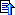robvolk
Most Valuable Yak

15732 PostsPosted - 2004-03-14 : 12:54:39 BRILLIANT!!!ditch
Master Smack Fu Yak Hacker

1466 PostsPosted - 2004-03-15 : 05:26:58 quote:Originally posted by MerkinROFLMAOThanks Guys.What does ROFLMAO Mean?Duane.Frank Kalis
Constraint Violating Yak Guru

413 PostsPosted - 2004-03-15 : 06:14:19 http://www.acronymfinder.com/af-query.asp?String=exact&Acronym=ROFLMAO&Find=Find--Frankhttp://www.insidesql.deditch
Master Smack Fu Yak Hacker

1466 PostsPosted - 2004-03-15 : 06:20:17 Thanks FrankDuane.Frank Kalis
Constraint Violating Yak Guru

413 PostsPosted - 2004-03-15 : 06:23:34 No problem!What about offering this to Microsoft as future enhancement by putting it on the wish list?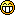--Frankhttp://www.insidesql.deharshal_in
Aged Yak Warrior

633 PostsPosted - 2004-03-15 : 06:26:41 Simply GREAT!!He is a fool for five minutes who asks , but who does not ask remains a fool for life!mohdowais
Sheikh of Yak Knowledge

1456 PostsPosted - 2004-03-15 : 06:55:52 quote:Originally posted by Frank KalisNo problem!What about offering this to Microsoft as future enhancement by putting it on the wish list?--Frankhttp://www.insidesql.dehuh? The world's already dividing over the inclusion of an analog clock in Longhornhttp://journals.tuxreports.com/lch/archives/000991.htmlhttp://weblogs.asp.net/oldnewthing/archive/2003/10/30/55498.aspxOSFrank Kalis
Constraint Violating Yak Guru

413 PostsPosted - 2004-03-15 : 07:04:08Yes, and I heard that M\$ once again teamed up with leading hardware vendors to finally supply a secure PC. This is a top secret document from the test labs in Denmarkhttp://www.uoe.dk/csworld/security-.html--Frankhttp://www.insidesql.detkizer
Almighty SQL Goddess

38200 PostsPosted - 2004-03-15 : 16:46:53 http://weblogs.sqlteam.com/tarad/archive/2004/03/15/1060.aspxTaraSamC
White Water Yakist

3467 PostsPosted - 2004-03-15 : 22:25:31 The frontiers of SQL are best explored by those with time on their hands. (What I mean is... I'm not sure myself. Go figure.)ditch
Master Smack Fu Yak Hacker

1466 PostsPosted - 2004-03-15 : 23:48:10 quote:Originally posted by tdugganhttp://weblogs.sqlteam.com/tarad/archive/2004/03/15/1060.aspxThanks for that Tara.Duane.anolis
Starting Member

14 PostsPosted - 2004-05-14 : 14:09:08 Great!!I've got a function that stores the current time in a table. When you create a job, it can do that every second. Anyone interested?Anolis,Developer,MCAD for .NETRatboy
Starting Member

2 PostsPosted - 2004-06-08 : 08:34:01 I just came across your post and was amazed to see that someone else out that was as bored as I was to create an analogue clock with the current time! If you're interested, here was my attempt:/** Init variables **/DECLARE @curX decimal(20, 10)DECLARE @curY decimal(20, 10)DECLARE @strLine varchar(100)DECLARE @intDistance intDECLARE @curA decimal(20, 10)DECLARE @curB decimal(20, 10)DECLARE @curC decimal(20, 10)DECLARE @curHourHandLen decimal(9, 4)DECLARE @curHourHandX1 decimal(20, 10)DECLARE @curHourHandY1 decimal(20, 10)DECLARE @curHourHandX2 decimal(20, 10)DECLARE @curHourHandY2 decimal(20, 10)DECLARE @curMinuteHandLen decimal(9, 4)DECLARE @curMinuteHandX1 decimal(20, 10)DECLARE @curMinuteHandY1 decimal(20, 10)DECLARE @curMinuteHandX2 decimal(20, 10)DECLARE @curMinuteHandY2 decimal(20, 10)DECLARE @curAngle numeric(11, 10)DECLARE @intQuarter smallintDECLARE @curHour decimal(20, 10)DECLARE @curDistanceFromCenter decimal(20, 10)DECLARE @curCenterX decimal(20, 10)DECLARE @curCenterY decimal(20, 10)/** Setup variables **/SET @curHourHandLen = 20SET @curMinuteHandLen = 42SET @curCenterX = 50SET @curCenterY = 50/** Work out the hand positions **/-- They all start from the centerSET @curHourHandX1 = @curCenterXSET @curHourHandY1 = @curCenterYSET @curMinuteHandX1 = @curCenterXSET @curMinuteHandY1 = @curCenterY-- Work out how far round the clock the hour is and set the position from thatSET @curHour = DATEPART(hour, GETDATE())IF @curHour > 12 SET @curHour = @curHour - 12SET @curHour = @curHour + (CONVERT(numeric(11, 9), DATEPART(minute, GETDATE())) / 60)SET @curAngle = (PI() * 2) * (CONVERT(numeric(11, 9), @curHour) / 12)IF @curAngle >= 0 AND @curAngle <= PI() / 2BEGIN SET @intQuarter = 1 SET @curHourHandX2 = @curHourHandX1 + (@curHourHandLen * SIN(@curAngle)) SET @curHourHandY2 = @curHourHandY1 - (@curHourHandLen * COS(@curAngle))ENDELSE IF @curAngle > PI() / 2 AND @curAngle <= PI()BEGIN SET @intQuarter = 2 SET @curAngle = PI() - @curAngle SET @curHourHandX2 = @curHourHandX1 + (@curHourHandLen * SIN(@curAngle)) SET @curHourHandY2 = @curHourHandY1 + (@curHourHandLen * COS(@curAngle))ENDELSE IF @curAngle > PI() AND @curAngle <= (PI() * 1.5)BEGIN SET @intQuarter = 3 SET @curAngle = @curAngle - PI() SET @curHourHandX2 = @curHourHandX1 - (@curHourHandLen * SIN(@curAngle)) SET @curHourHandY2 = @curHourHandY1 + (@curHourHandLen * COS(@curAngle))ENDELSE IF @curAngle > (PI() * 1.5) AND @curAngle <= (PI() * 2)BEGIN SET @intQuarter = 4 SET @curAngle = (PI() * 2) - @curAngle SET @curHourHandX2 = @curHourHandX1 - (@curHourHandLen * SIN(@curAngle)) SET @curHourHandY2 = @curHourHandY1 - (@curHourHandLen * COS(@curAngle))ENDELSEBEGIN PRINT 'Could not work out the hour hand''s quarter segment' PRINT @curAngle RETURNEND-- Work out how far round the clock the minute is and set the position from thatSET @curAngle = (PI() * 2) * (CONVERT(numeric(11, 9), DATEPART(minute, GETDATE())) / 60)IF @curAngle >= 0 AND @curAngle <= PI() / 2BEGIN SET @intQuarter = 1 SET @curMinuteHandX2 = @curMinuteHandX1 + (@curMinuteHandLen * SIN(@curAngle)) SET @curMinuteHandY2 = @curMinuteHandY1 - (@curMinuteHandLen * COS(@curAngle))ENDELSE IF @curAngle > PI() / 2 AND @curAngle <= PI()BEGIN SET @intQuarter = 2 SET @curAngle = PI() - @curAngle SET @curMinuteHandX2 = @curMinuteHandX1 + (@curMinuteHandLen * SIN(@curAngle)) SET @curMinuteHandY2 = @curMinuteHandY1 + (@curMinuteHandLen * COS(@curAngle))ENDELSE IF @curAngle > PI() AND @curAngle <= (PI() * 1.5)BEGIN SET @intQuarter = 3 SET @curAngle = @curAngle - PI() SET @curMinuteHandX2 = @curMinuteHandX1 - (@curMinuteHandLen * SIN(@curAngle)) SET @curMinuteHandY2 = @curMinuteHandY1 + (@curMinuteHandLen * COS(@curAngle))ENDELSE IF @curAngle > (PI() * 1.5) AND @curAngle <= (PI() * 2)BEGIN SET @intQuarter = 4 SET @curAngle = (PI() * 2) - @curAngle SET @curMinuteHandX2 = @curMinuteHandX1 - (@curMinuteHandLen * SIN(@curAngle)) SET @curMinuteHandY2 = @curMinuteHandY1 - (@curMinuteHandLen * COS(@curAngle))ENDELSEBEGIN PRINT 'Could not work out the minute hand''s quarter segment' PRINT @curAngle RETURNEND/** Loop through Y axis **/SET @curY = 0WHILE @curY <= 100BEGIN /** Loop through X axis **/ SET @curX = 0 SET @strLine = '' WHILE @curX <= 100 BEGIN /** Need to work out how far away the coordinate is from the hour hand **/ -- Find lengths A, B and C for triangle made by hour hand's line and the coordinate -- C is the hour hand, coordinate is inbetween A and B SET @curA = SQRT(POWER(ABS(@curX - @curHourHandX1), 2) + POWER(ABS(@curY - @curHourHandY1), 2)) SET @curB = SQRT(POWER(ABS(@curHourHandX2 - @curX), 2) + POWER(ABS(@curHourHandY2 - @curY), 2)) SET @curC = SQRT(POWER(ABS(@curHourHandX2 - @curHourHandX1), 2) + POWER(ABS(@curHourHandY2 - @curHourHandY1), 2)) IF @curB = 0 OR @curC = 0 SET @curAngle = 0 ELSE SET @curAngle = (POWER(@curB, 2) + POWER(@curC, 2) - POWER(@curA, 2)) / (2 * @curB * @curC) SET @curAngle = ACOS(@curAngle) -- If coordinate is perpendicular to line, get the length of the perpendicular -- otherwise the length from the coordinate to the end of the line IF @curA * COS(@curAngle) < 0 OR @curA * COS(@curAngle) > @curHourHandLen SET @intDistance = @curA ELSE IF @curB * COS(@curAngle) < 0 OR @curB * COS(@curAngle) > @curHourHandLen SET @intDistance = @curB ELSE SET @intDistance = CONVERT(int, @curB * SIN(@curAngle)) /** Need to work out how far away the coordinate is from the minute hand **/ -- Find lengths A, B and C for triangle made by minute hand's line and the coordinate -- C is the minute hand, coordinate is inbetween A and B SET @curA = SQRT(POWER(ABS(@curX - @curMinuteHandX1), 2) + POWER(ABS(@curY - @curMinuteHandY1), 2)) SET @curB = SQRT(POWER(ABS(@curMinuteHandX2 - @curX), 2) + POWER(ABS(@curMinuteHandY2 - @curY), 2)) SET @curC = SQRT(POWER(ABS(@curMinuteHandX2 - @curMinuteHandX1), 2) + POWER(ABS(@curMinuteHandY2 - @curMinuteHandY1), 2)) IF @curB = 0 OR @curC = 0 SET @curAngle = 0 ELSE SET @curAngle = (POWER(@curB, 2) + POWER(@curC, 2) - POWER(@curA, 2)) / (2 * @curB * @curC) SET @curAngle = ACOS(@curAngle) -- If coordinate is perpendicular to line, get the length of the perpendicular -- otherwise the length from the coordinate to the end of the line IF @curA * COS(@curAngle) < 0 OR @curA * COS(@curAngle) > @curMinuteHandLen BEGIN IF @curA < @intDistance SET @intDistance = CONVERT(int, @curA) END ELSE IF @curB * COS(@curAngle) < 0 OR @curB * COS(@curAngle) > @curMinuteHandLen BEGIN IF @curB < @intDistance SET @intDistance = CONVERT(int, @curB) END ELSE IF CONVERT(int, @curB * SIN(@curAngle)) < @intDistance SET @intDistance = CONVERT(int, @curB * SIN(@curAngle)) /** Finally add a circle round the whole thing **/ SET @curDistanceFromCenter = SQRT(POWER(ABS(@curX - @curCenterX), 2) + POWER(ABS(@curY - @curCenterY), 2)) IF ABS(CONVERT(int, @curDistanceFromCenter - 46)) < @intDistance SET @intDistance = CONVERT(int, @curDistanceFromCenter - 46) /** Find out what's at this coordinate **/ IF @curX = 50 AND @curY = 50 SET @strLine = @strLine + 'O' else SET @strLine = @strLine + CASE @intDistance WHEN 0 THEN '#' WHEN 1 THEN ':' WHEN 2 THEN '·' ELSE ' ' END SET @curX = @curX + 1 --if @intdistance = 0 then set @curtemp = @curAngle END PRINT @strLine SET @curY = @curY + 1ENDditch
Master Smack Fu Yak Hacker

1466 PostsPosted - 2004-06-08 : 09:08:53 Nice OneGreat minds think alike heyDuane.Ratboy
Starting Member

2 PostsPosted - 2004-06-08 : 09:29:50 True - I reckon if we work together we could get a radio alarm going too...maya_zakry
Constraint Violating Yak Guru

379 PostsPosted - 2007-06-15 : 04:58:58 just found this link.. this is really kewl.. how could someone bored came out witnh this.. ahhaha~~~Focus on problem, not solution ¯\(º_o)/¯ ~~~ditch
Master Smack Fu Yak Hacker

1466 PostsPosted - 2013-07-22 : 15:20:31 OK - so I was bored again and decided to upgrade this to use the built-in CLR GEOMETRY functionality.To see the results.Click on the spatial results tab when completed.`DECLARE @ClockRadius INTDECLARE @PivotX INTDECLARE @PivotY INTDECLARE @Angle DECIMAL(5, 2)DECLARE @GeomString VARCHAR(MAX) DECLARE @GeomStringHH VARCHAR(MAX)DECLARE @GeomStringMin VARCHAR(MAX)DECLARE @Hours INTDECLARE @Minutes INT SET @ClockRadius = 200SET @PivotX = @ClockRadius + 10SET @PivotY = @ClockRadius + 10CREATE TABLE #AngleMaster( theAngle DECIMAL(5,2), theHour DECIMAL(5, 2), theMinute DECIMAL(5, 2))CREATE TABLE #Draw( OrderBy INT IDENTITY(1, 1), X INT, Y INT)SET @Angle = 0WHILE @Angle <= 360BEGIN INSERT INTO #AngleMaster ( theAngle, theHour, theMinute ) VALUES ( @Angle, CASE WHEN @Angle > 270 THEN @Angle / 30.00 - 9 ELSE @Angle / 30.00 + 3 END, CASE WHEN @Angle > 270 THEN @Angle / 6.00 - 45 ELSE @Angle / 6.00 + 15 END ) SET @Angle = @Angle + 0.5ENDINSERT INTO #DrawSELECT @PivotX + COS(RADIANS(theAngle)) * @ClockRadius AS X, @PivotY + SIN(RADIANS(theAngle)) * @ClockRadius AS YFROM #AngleMasterORDER BY theAngle INSERT INTO #DrawSELECT @PivotX + COS(RADIANS(theAngle)) * (@ClockRadius - 1) AS X, @PivotY + SIN(RADIANS(theAngle)) * (@ClockRadius - 1) AS YFROM #AngleMasterORDER BY theAngle INSERT INTO #DrawSELECT @PivotX + COS(RADIANS(theAngle)) * (@ClockRadius - 2) AS X, @PivotY + SIN(RADIANS(theAngle)) * (@ClockRadius - 2) AS YFROM #AngleMasterORDER BY theAngle INSERT INTO #DrawSELECT @PivotX + COS(RADIANS(theAngle)) * (@ClockRadius - 3) AS X, @PivotY + SIN(RADIANS(theAngle)) * (@ClockRadius - 3) AS YFROM #AngleMasterORDER BY theAngle INSERT INTO #DrawSELECT @PivotX + COS(RADIANS(theAngle)) * (@ClockRadius - 4) AS X, @PivotY + SIN(RADIANS(theAngle)) * (@ClockRadius - 4) AS YFROM #AngleMasterORDER BY theAngle SET @GeomString = ''SELECT @GeomString += STR(X, 3) + ' ' + STR(Y, 3) + ', 'FROM #Draw ORDER BY OrderBy --****Now get time and draw hands.SELECT @Hours = DATEPART(hh, GETDATE()), @Minutes = DATEPART(MINUTE, GETDATE()) SELECT @GeomStringHH = STR(@PivotX, 3) + ' ' + STR(@PivotY, 3) + ', ' + STR(@PivotX + 30, 3) + ' ' + STR(@PivotY + 30, 3) + ', ' + STR(@PivotX + 30 + COS(RADIANS(theAngle)) * (@ClockRadius * 0.6),3) + ' ' + STR(@PivotY + 30 - SIN(RADIANS(theAngle)) * (@ClockRadius * 0.6),3) + ', ' + STR(@PivotX + COS(RADIANS(theAngle)) * (@ClockRadius * 0.6),3) + ' ' + STR(@PivotY - SIN(RADIANS(theAngle)) * (@ClockRadius * 0.6),3) + ', ' + STR(@PivotX, 3) + ' ' + STR(@PivotY, 3) + ', 'FROM #AngleMaster WHERE theHour = CASE WHEN @Hours > 12 THEN @Hours - 12 ELSE @Hours ENDSELECT @GeomStringMin = STR(@PivotX, 3) + ' ' + STR(@PivotY, 3) + ', ' + STR(@PivotX + 10, 3) + ' ' + STR(@PivotY + 10, 3) + ', ' + STR(@PivotX + 10 + COS(RADIANS(theAngle)) * (@ClockRadius * 0.9),3) + ' ' + STR(@PivotY + 10 - SIN(RADIANS(theAngle)) * (@ClockRadius * 0.9),3) + ', ' + STR(@PivotX + COS(RADIANS(theAngle)) * (@ClockRadius * 0.9),3) + ' ' + STR(@PivotY - SIN(RADIANS(theAngle)) * (@ClockRadius * 0.9),3) + ', ' + STR(@PivotX, 3) + ' ' + STR(@PivotY, 3) + ', 'FROM #AngleMaster WHERE theMinute = @Minutes SELECT @GeomString = LEFT(@GeomString, LEN(@GeomString) - 1) --Get rid of last ', 'SELECT @GeomStringHH = LEFT(@GeomStringHH, LEN(@GeomStringHH) - 1) --Get rid of last ', 'SELECT @GeomStringMin = LEFT(@GeomStringMin, LEN(@GeomStringMin) - 1) --Get rid of last ', ' select @GeomStringMin SELECT geometry::STGeomFromText('LINESTRING (' + @GeomString + ')', 0)UNION ALLSELECT geometry::STGeomFromText('POLYGON ((' + @GeomStringHH + '))', 0)UNION ALLSELECT geometry::STGeomFromText('POLYGON ((' + @GeomStringMin + '))', 0) `I have also posted it on my blog here: http://ditchiecubeblog.wordpress.com/2013/07/22/time-in-analog-using-the-built-in-geometry-clr-functionality-in-sql-server-2/Duane.http://ditchiecubeblog.wordpress.com/xiaomingzc
Starting Member

3 PostsPosted - 2013-08-03 : 03:18:10 unspammed